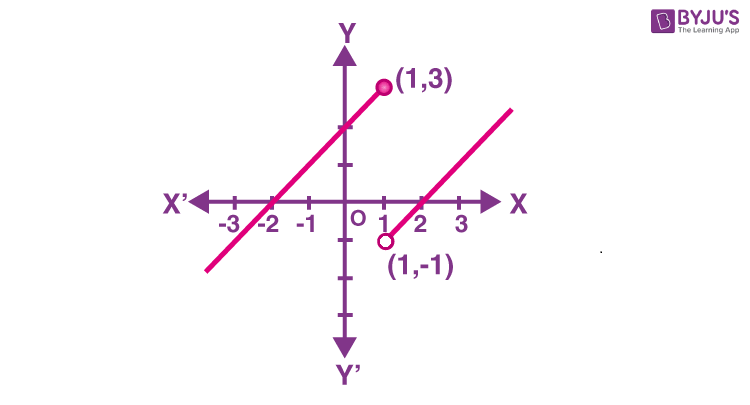# Continuous Function

In mathematics, a continuous function is a function that does not have discontinuities that means any unexpected changes in value. A function is continuous if we can ensure arbitrarily small changes by restricting enough minor changes in its input. If the given function is not continuous, then it is said to be discontinuous. In other words, we can say that a function is continuous at a fixed point if we can draw the graph of the function around that point without lifting the pen from the paper’s plane.

## Continuous Function Definition

Mathematically, we can define the continuous function using limits as given below:

Suppose f is a real function on a subset of the real numbers and let c be a point in the domain of f. Then f is continuous at c if

$$\begin{array}{l}\LARGE \lim_{x\rightarrow c}f(x)=f(c)\end{array}$$

We can elaborate the above definition as, if the left-hand limit, right-hand limit, and the function’s value at x = c exist and are equal to each other, the function f is continuous at x = c. If the right hand and left-hand limits at x = c coincide, then we can say that the expected value is the limit of the function at x = c. Hence, we may also rephrase the definition of continuity as “a function is continuous at x = c if the function is defined at x = c and if the function’s value at x = c equals the limit of the function at x = c”. If f is not continuous at c, then we can say that f is discontinuous at c and c is called a point of discontinuity of the given function f.

The other way of defining the continuous function is given below.

A real function f is continuous if it is continuous at every point in the domain of f. We can explain this in detail with mathematical terms as:

Suppose f is a function defined on a closed interval [a, b], then for f to be continuous, it needs to be continuous at every point in [a, b], including the endpoints a and b.

Continuity of f(x) at a means

$$\begin{array}{l}\lim_{x\rightarrow a^+}f(x)=f(a)\end{array}$$

and continuity of f(x) at b means

$$\begin{array}{l}\lim_{x\rightarrow b^-}f(x)=f(b)\end{array}$$

Thus, if f is defined only at one point, it is continuous there, i.e., if the domain of f is a singleton, the function f will be a continuous function.

From the above definitions, we can define three conditions to check the continuity of the given function. They are:

Consider the function f(x) and point x = a.

1. The function must be defined at a point a to be continuous at that point x = a.

2. The limit of the function f(x) should be defined at the point x = a,

3. The value of the function f(x) at that point, i.e. f(a) must equal the value of the limit of f(x) at x = a.

Let’s have a look at the examples given below to understand how to check the continuity of the given function at a point.

## Continuous Function Examples

Example 1:

Check the continuity of the function f given by f(x) = 3x + 2 at x = 1.

Solution:

Given,

f(x) = 3x + 2

Substituting x = 1 in f(x),

f(1) = 3(1) + 2 = 3 + 2 = 5

Thus, the function is defined at the given point x = 1 and its value is 5.

Now, we have to find the limit of the function at x = 1.

$$\begin{array}{l}\lim_{x\rightarrow 1}f(x)=\lim_{x\rightarrow 1}(3x + 2) = 3(1)+2 = 5 = f(1)\end{array}$$

Therefore, the given function is continuous at x = 1.

Example 2:

Check whether the given function is continuous.

$$\begin{array}{l}f(x)=\left\{\begin{matrix} x+2; &x\le 1 \\ x-2; & x>1 \end{matrix}\right.\end{array}$$

Solution:

Given,

$$\begin{array}{l}f(x)=\left\{\begin{matrix} x+2; &x\le 1 \\ x-2; & x>1 \end{matrix}\right.\end{array}$$

This function is defined for all the points of the real line.

Let’s check the continuity of the given function in different cases.

Case 1:

When x < 1,

f(x) = x + 2

Consider c < 1 and f(c) = c + 2

Now,

$$\begin{array}{l}\lim_{x \rightarrow c}f(x) = \lim_{x \rightarrow c}x+2 = c+2\end{array}$$

Thus, the function f(x) is continuous at all real numbers less than 1.

Case 2:

When x > 1

f(x) = x – 2

Consider c > 1 and f(c) = c – 2

$$\begin{array}{l}\lim_{x \rightarrow c}f(x) = \lim_{x \rightarrow c}x-2 = c-2\end{array}$$

Thus, the function f(x) is continuous at all real numbers greater than 1.

Case 3:

When x = 1, f(x) = x + 2

Consider c = 1, now we have to find the left-hand and right-hand limits.

LHL

$$\begin{array}{l}\lim_{x \rightarrow 1^-}f(x) = \lim_{x \rightarrow 1^-}x+2 = 1+2=3\end{array}$$

RHL

$$\begin{array}{l}\lim_{x \rightarrow 1^+}f(x) = \lim_{x \rightarrow 1^+}x-2 = 1-2=-1\end{array}$$

Here, the left-hand limit is not equal to the right-hand limit.

Thus, the function f(x) is not continuous at x = 1.

Hence, x = 1 is the only point of discontinuity of f.

### Continuous Function Graph

We can represent the continuous function using graphs. For the example 2 (given above), we can draw the graph as given below:In this graph, we can clearly see that the function is not continuous at x = 1.

However, it is easy to conclude whether the given graph is of a continuous or discontinuous function.